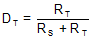SBOS710D October   2014  – February 2018

PRODUCTION DATA.

1. Features
2. Applications
3. Description
4. Revision History
5. Pin Configuration and Functions
6. Specifications
7. Parameter Measurement Information
8. Detailed Description
1. 8.1 Overview
2. 8.2 Functional Block Diagram
3. 8.3 Feature Description
4. 8.4 Device Functional Modes
9. Application and Implementation
1. 9.1 Application Information
2. 9.2 Typical Application
1. 9.2.1 Design Requirements
2. 9.2.2 Detailed Design Procedure
3. 9.2.3 Application Curves
3. 9.3 Do's and Don'ts
10. 10Power Supply Recommendations
11. 11Layout
12. 12Device and Documentation Support
13. 13Mechanical, Packaging, and Orderable Information

• RMS|14

#### 9.1.4 Noise Figure

Noise figure (NF) is a helpful measurement in an RF system design. The basis of this calculation is to define how much thermal noise the system (or even on the component) adds to this input signal. All systems are assumed to have a starting thermal noise power of –174 dBm/√Hz at room temperature calculated from P(dBW) = 10 × log (kTB), where T is temperature in Kelvin (290k), B is bandwidth in Hertz (1 Hz), and k is Boltzmann's constant 1.38 × 10–23 (J / K). Whenever an element is placed in a system, additional noise is added beyond the thermal noise floor. The noise factor (NF) helps calculate the noise figure and is the ratio between the input SNR and the output SNR. Input SNR includes the noise contribution from the resistive part of the source impedance, ZS. NF is relative to the source impedance used in the measurement or calculation because capacitors and inductors are known to be noiseless. Equation 9 calculates NF:

Equation 9. NF = 10 log (eno2 / enZs)

where

• en(Zs) is the thermal noise of the source resistance and equal to 4 kTRS (GDT)2,
• G is the voltage gain of the amplifier.

From Equation 10, NF is approximately equal to 10 dB which is the just above the actual value of 9.6 dB measured on the bench at 200 MHz when referenced to 50 Ω and as shown in Figure 59.

Equation 10.For thermal noise calculations with different source resistances, Equation 11 can be used to calculate the NF change with a new source resistance. For example, Equation 9 uses a source resistance of 50 Ω. By using a source of 100 Ω, the new noise figure calculation (Equation 11) yields an NF of 6.6 dB, which provides a 3-dB improvement that results from the increase in Zs.

Equation 11. en(Zs) = kTRs